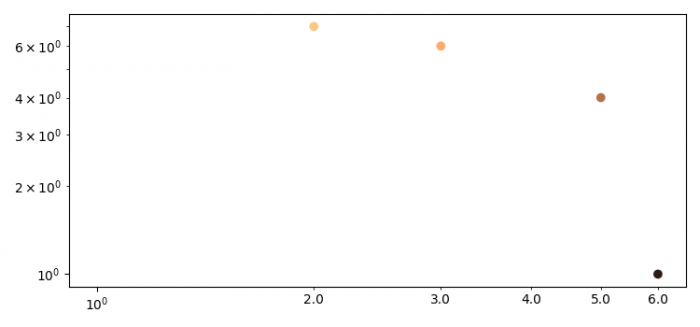# How to remove scientific notation from a Matplotlib log-log plot?

To remove scientific notation from a matplotlib log-log plot, we can use ax.xaxis.set_minor_formatter(mticker.ScalarFormatter()) statement.

## Steps

• Set the figure size and adjust the padding between and around the subplots.
• Create x and y data points using numpy.
• Plot x and y data points using scatter() method.
• Set x and y axes sacle using set_xscale() and set_yscale() methods.
• To remove scientific notation, use format tick values as a number.
• To display the figure, use show() method.

## Example

import numpy as np
from matplotlib import pyplot as plt, ticker as mticker

plt.rcParams["figure.figsize"] = [7.50, 3.50]
plt.rcParams["figure.autolayout"] = True

x = np.array([1, 7, 6, 4, 0])
y = np.array([6, 2, 3, 5, 1])

plt.scatter(y, x, c=x, cmap="copper")

ax = plt.gca()
ax.set_xscale('log')
ax.set_yscale('log')
ax.xaxis.set_minor_formatter(mticker.ScalarFormatter())

plt.show()

## Output